Published: 27 December 2022

# Model of a pipe robot with limited interactions

K. Ragulskis1
B. Spruogis2
A. Matuliauskas3
V. Mištinas4
L. Ragulskis5
1Kaunas University of Technology, K. Donelaičio str. 73, LT-44249, Kaunas, Lithuania
2, 3, 4Department of Mobile Machinery and Railway Transport, Faculty of Transport Engineering, Vilnius Gediminas Technical University, Plytinės str. 27, LT-10105, Vilnius, Lithuania
5Department of Systems Analysis, Faculty of Informatics, Vytautas Magnus University, Vileikos str. 8, LT-44404, Kaunas, Lithuania
Corresponding Author:
K. Ragulskis
Views 73

#### Abstract

Nonlinear interactions between the exciting mass and the case of a pipe robot are important in order to prevent impacts of the exciting mass with the case of the pipe robot. Those impacts lead to deterioration of operation of a pipe robot and even may lead to destruction of some parts of the robot. Model for the analysis of dynamics of a pipe robot with limited interactions is proposed in this paper. For this purpose, a special expression of nonlinear stiffness is used. Results of investigations for various parameters of the system are presented.

## 1. Introduction

Nonlinear interactions between the exciting mass and the case of a pipe robot are important in order to prevent impacts of the exciting mass with the case of the pipe robot. Those impacts lead to deterioration of operation of a pipe robot and even may lead to destruction of some parts of the robot.

Model for the analysis of dynamics of a pipe robot with limited interactions is proposed in this paper. For this purpose, a special expression of nonlinear stiffness is used. Results of investigations for various parameters of the system are presented.

Resonances of dynamical systems are investigated in . Impact motions are analyzed in . Stabilization of vibrating systems is investigated in . Vibrations and impacts are analyzed in . Periodic orbits of dynamical systems are investigated in . Energy sink of vibro-impact type is analyzed in . Impact of a particle with a wall is investigated in . Investigation of frequencies of a dynamical system is presented in . Dynamics of a pendulum is analyzed in . System with piecewise linearity is investigated in . Vibrating system with resonant zones is analyzed in . Investigation of the Sommerfeld effect is described in . Dynamical systems with isolated resonances are analyzed in .

Similar model of a pipe robot without interactions of limited displacement type is investigated in . Main objective of this paper is to investigate the effect of interactions of limited displacement type to the dynamic behavior of a pipe robot.

Model of a pipe robot with two degrees of freedom and nonlinear interactions of limited relative displacement type is described. Then results of numerical investigations for various parameters of the investigated pipe robot are presented.

## 2. The model of a system with limited displacements

The system is described by the equation:

1
${x}^{\text{'}\text{'}}+2h{x}^{\text{'}}+\frac{1}{1-\left|x\right|}x=f\mathrm{s}\mathrm{i}\mathrm{n}\nu \tau ,$

where $x$ denotes the displacement, $h$ denotes the coefficient of viscous friction, $f$ denotes the amplitude of excitation, $\nu$ denotes the frequency of excitation, $\tau$ denotes the time and prime denotes differentiation with respect to the time.

### 2.1. Conservative model when $\mathbit{h}=0$, $\mathbit{f}=0$

Results for $x\left(0\right)=0$, $x\text{'}\left(0\right)=1$ are shown in Fig. 1. Results for $x\left(0\right)=0$, $x\text{'}\left(0\right)=$ 1.5 are shown in Fig. 2.

For greater value of initial velocity, the effect of nonlinear stiffness is greater, and this is clearly seen from the dependence of force of stiffness from displacement.

Fig. 1Conservative system for x0=0, x'0=1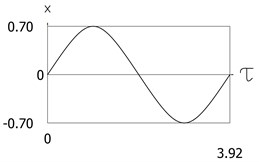a) Time history of displacement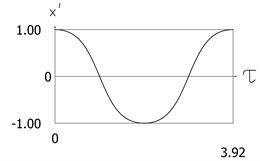b) Time history of velocity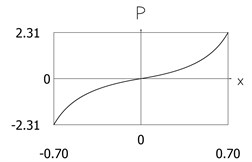c) Dependence of force of stiffness from displacement

Fig. 2Conservative system for x0=0, x'0=1.5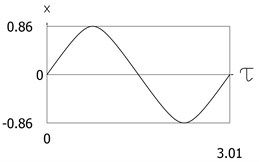a) Time history of displacementb) Time history of velocity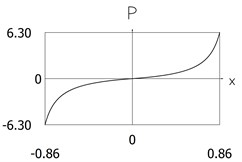c) Dependence of force of stiffness from displacement

### 2.2. Amplitude frequency characteristics of the conservative model

Amplitude frequency characteristics for the displacement and for the velocity are shown in Fig. 3.

Hardening effect is seen from the presented results. For higher nonlinearity the increase of the amplitude of the third harmonic is seen.

Fig. 3Amplitude frequency characteristics: constant part and amplitudes of the first three harmonics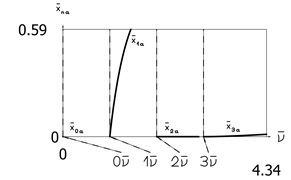a) Displacement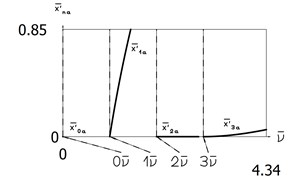b) Velocity

### 2.3. Dynamics of the forced dissipative model

The parameters of the system are set to $h=0.1$, $f=1.$ Steady state solutions are depicted in Fig. 4 and in Fig. 5.

Fig. 4Steady state motion for h=0.1, f=1, ν=1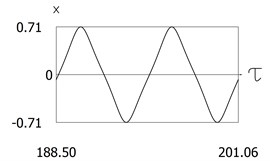a) Time history of displacement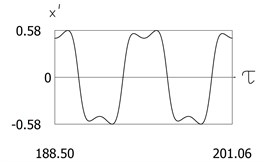b) Time history of velocity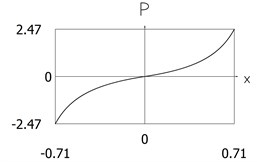c) Dependence of force of stiffness from displacement

Fig. 5Steady state motion for h=0.1, f=1, ν=1.5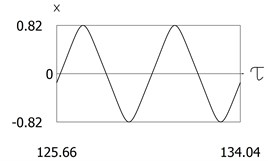a) Time history of displacement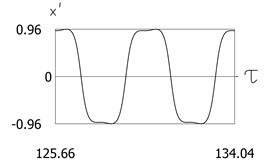b) Time history of velocity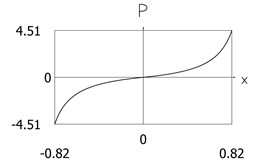c) Dependence of force of stiffness from displacement

For higher value of frequency of excitation, the nonlinear effect is greater, and this is clearly seen from the dependence of the force of stiffness from the displacement.

## 3. The model of a pipe robot with limited interactions

The schematic diagram of the pipe robot is depicted in Fig. 6.

Fig. 6Principle of operation of a pipe robot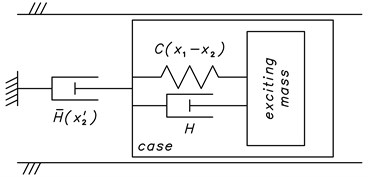The investigated system has two degrees of freedom and is described by the two differential equations:

2
${x\text{'}\text{'}}_{1}+h\left({x\text{'}}_{1}-{x\text{'}}_{2}\right)+\frac{1}{1-\left|{x}_{1}-{x}_{2}\right|}\left({x}_{1}-{x}_{2}\right)={f}_{0}\mathrm{s}\mathrm{i}\mathrm{n}\nu \tau ,$
3
$\mu {x\text{'}\text{'}}_{2}+h\left({x\text{'}}_{2}-{x\text{'}}_{1}\right)+\frac{1}{1-\left|{x}_{1}-{x}_{2}\right|}\left({x}_{2}-{x}_{1}\right)+\left\{\begin{array}{l}{h}_{1}{x\text{'}}_{2},\mathrm{}\mathrm{w}\mathrm{h}\mathrm{e}\mathrm{n}\mathrm{}{x\text{'}}_{2}>0\\ {h}_{2}{x\text{'}}_{2},\mathrm{}\mathrm{w}\mathrm{h}\mathrm{e}\mathrm{n}\mathrm{}{x\text{'}}_{2}<0\end{array}\right\}=0,$

where ${x}_{1}$ denotes the displacement of the exciting mass located inside of the pipe robot, ${x}_{2}$ denotes the displacement of the case of the pipe robot, $\mu$ denotes the mass of the case of the investigated pipe robot, $h$ denotes the coefficient of viscous friction between the exciting mass located inside of the pipe robot and the case of the investigated pipe robot, ${h}_{1}$ denotes the coefficient of viscous friction of the case of the investigated pipe robot with respect to the pipe for positive velocity of motion of the investigated pipe robot, ${h}_{2}$ denotes the coefficient of viscous friction of the case of the investigated pipe robot with respect to the pipe for negative velocity of motion of the investigated pipe robot, ${f}_{0}$ denotes the amplitude of excitation, $\nu$ denotes the frequency of excitation, $\tau$ denotes the time, and prime denotes differentiation with respect to the time.

It was assumed that the parameters of the investigated vibrating system have the following values: $\mu =0.1$, $h=0.1$, ${h}_{1}=0.2$, $\nu =1.$ Investigations for two values of amplitude of excitation were performed at ${f}_{0}=1$ and ${f}_{0}=10.$ Also, investigations for two values of viscous friction of the case of the investigated pipe robot with respect to the pipe for negative velocity of motion of the investigated pipe robot were performed at ${h}_{2}=0.1$ and ${h}_{2}=2.$ Calculations from zero initial conditions were performed: ${x}_{1}\left(0\right)=0$, ${x}_{2}\left(0\right)=0$, ${x\text{'}}_{1}\left(0\right)=0$, ${x\text{'}}_{2}\left(0\right)=0.$

In order to visually estimate that the steady state regime has been reached two periods of steady state motions are represented.

## 4. Dynamics of the proposed model of a pipe robot with limited interactions

### 4.1.1. Dynamics of the pipe robot with limited interactions for ${\mathbit{h}}_{2}=0.1$

Displacement of the first degree of freedom, velocity of the first degree of freedom, displacement of the second degree of freedom, velocity of the second degree of freedom as functions of time are shown in Fig. 7.

Relative displacement and relative velocity as functions of time are shown in Fig. 8.

Fig. 7Dynamics of the pipe robot with limited interactions for μ=0.1, h=0.1, h1=0.2, ν=1, f0=1, h2=0.1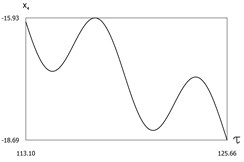a) Displacement of the first degree of freedom as function of time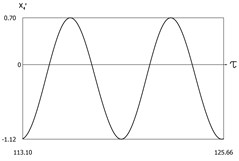b) Velocity of the first degree of freedom as function of time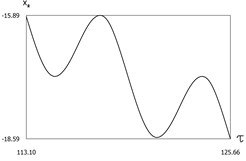c) Displacement of the second degree of freedom as function of time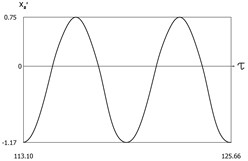d) Velocity of the second degree of freedom as function of time

Fig. 8Relative motions in the pipe robot with limited interactions for μ=0.1, h=0.1, h1=0.2, ν=1, f0=1, h2=0.1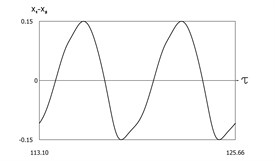a) Relative displacement as function of time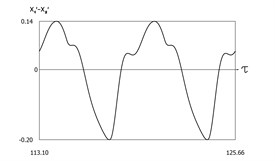b) Relative velocity as function of time

### 4.1.2. Dynamics of the pipe robot with limited interactions for ${\mathbit{h}}_{2}=2$

Displacement of the first degree of freedom, velocity of the first degree of freedom, displacement of the second degree of freedom, velocity of the second degree of freedom as functions of time are shown in Fig. 9.

Fig. 9Dynamics of the pipe robot with limited interactions for μ=0.1, h=0.1, h1=0.2, ν=1, f0=1, h2=2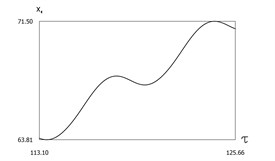a) Displacement of the first degree of freedom as function of time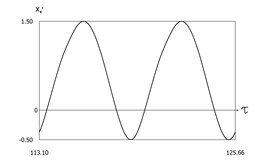b) Velocity of the first degree of freedom as function of time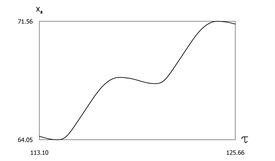c) Displacement of the second degree of freedom as function of time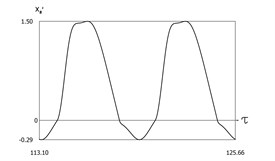d) Velocity of the second degree of freedom as function of time

Relative displacement and relative velocity as functions of time are shown in Fig. 10.

Fig. 10Relative motions in the pipe robot with limited interactions for μ=0.1, h=0.1, h1=0.2, ν=1, f0=1, h2=2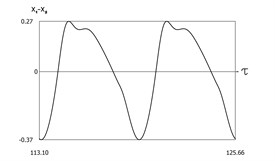a) Relative displacement as function of time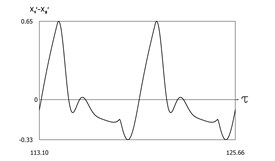b) Relative velocity as function of time

From the presented results it is seen that for the first value of viscous friction of the case of the investigated pipe robot with respect to the pipe for negative velocity of motion of the investigated pipe robot motion of the pipe robot in the negative direction of the $x$ axis is observed, while for the second value of viscous friction of the case of the investigated pipe robot with respect to the pipe for negative velocity of motion of the investigated pipe robot motion of the pipe robot in the positive direction of the $x$ axis is observed.

### 4.2.1. Dynamics of the pipe robot with limited interactions for ${\mathbit{h}}_{2}=0.1$

Displacement of the first degree of freedom, velocity of the first degree of freedom, displacement of the second degree of freedom, velocity of the second degree of freedom as functions of time are shown in Fig. 11.

Fig. 11Dynamics of the pipe robot with limited interactions for μ=0.1, h=0.1, h1=0.2, ν=1, f0=10, h2=0.1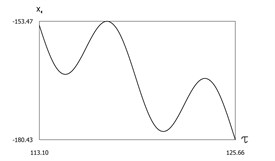a) Displacement of the first degree of freedom as function of time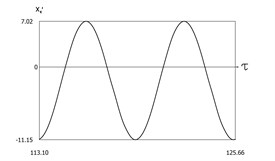b) Velocity of the first degree of freedom as function of time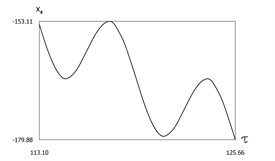c) Displacement of the second degree of freedom as function of time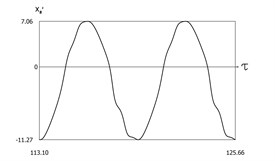d) Velocity of the second degree of freedom as function of time

Relative displacement and relative velocity as functions of time are shown in Fig. 12.

Fig. 12Relative motions in the pipe robot with limited interactions for μ=0.1, h=0.1, h1=0.2, ν=1, f0=10, h2=0.1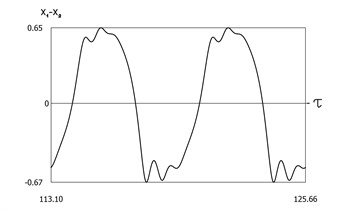a) Relative displacement as function of time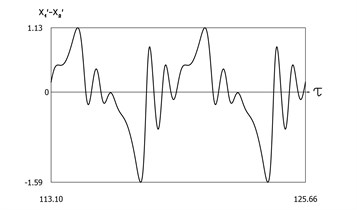b) Relative velocity as function of time

### 4.2.2. Dynamics of the pipe robot with limited interactions for ${\mathbit{h}}_{2}=2$

Displacement of the first degree of freedom, velocity of the first degree of freedom, displacement of the second degree of freedom, velocity of the second degree of freedom as functions of time are shown in Fig. 13.

Relative displacement and relative velocity as functions of time are shown in Fig. 14.

From the presented results it is seen that for the first value of viscous friction of the case of the investigated pipe robot with respect to the pipe for negative velocity of motion of the investigated pipe robot motion of the pipe robot in the negative direction of the $x$ axis is observed, while for the second value of viscous friction of the case of the investigated pipe robot with respect to the pipe for negative velocity of motion of the investigated pipe robot motion of the pipe robot in the positive direction of the $x$ axis is observed.

From the obtained results it can be seen that for the case when amplitude of excitation is high, the distance travelled by the investigated pipe robot with limited interactions is much greater than for the case when amplitude of excitation is low.

Similar model of a pipe robot without interactions of limited displacement type is investigated in . From the results presented in this paper the effect of interactions of limited displacement type to the dynamic behavior of a pipe robot can be seen.

Fig. 13Dynamics of the pipe robot with limited interactions for μ=0.1, h=0.1, h1=0.2, ν=1, f0=10, h2=2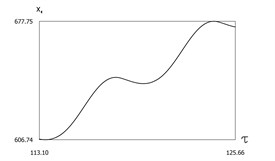a) Displacement of the first degree of freedom as function of time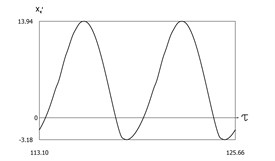b) Velocity of the first degree of freedom as function of time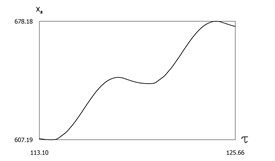c) Displacement of the second degree of freedom as function of time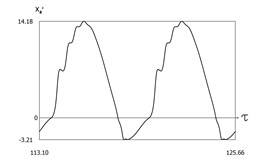d) Velocity of the second degree of freedom as function of time

Fig. 14Relative motions in the pipe robot with limited interactions for μ=0.1, h=0.1, h1=0.2, ν=1, f0=10, h2=2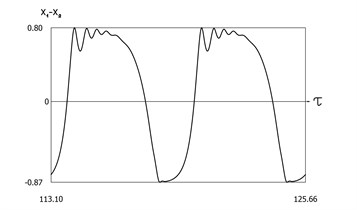a) Relative displacement as function of time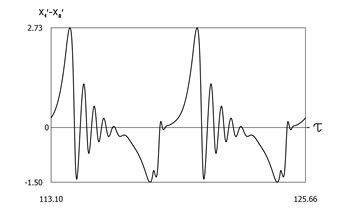b) Relative velocity as function of time

## 5. Conclusions

Pipe robot with limited interactions is investigated. Nonlinear interactions between the exciting mass and the case of a pipe robot are important in order to prevent impacts of the exciting mass with the case of the pipe robot. Those impacts lead to deterioration of operation of a pipe robot and even may lead to destruction of some parts of the robot. Model for the analysis of dynamics of a pipe robot with two degrees of freedom and nonlinear interactions of limited relative displacement type is proposed in this paper. For this purpose, a special expression of nonlinear stiffness is used.

Results of numerical investigations for various parameters of the investigated pipe robot are presented. From the presented results it is seen that depending on the value of viscous friction of the case of the investigated pipe robot with respect to the pipe for negative velocity of motion of the investigated pipe robot motion of the pipe robot in the negative direction of the $x$ axis can be observed as well as motion of the pipe robot in the positive direction of the $x$ axis can be observed.

Also, from the obtained results it can be seen that for the case when amplitude of excitation is high, the distance travelled by the investigated pipe robot with limited interactions is much greater than for the case when amplitude of excitation is low.

The presented results can be used in the design of pipe robots with limited interactions.

19 September 2022
Accepted
20 December 2022
Published
27 December 2022
Keywords
pipe robot
limited interactions
nonlinear stiffness
Acknowledgements

The authors have not disclosed any funding.

Data Availability

The datasets generated during and/or analyzed during the current study are available from the corresponding author on reasonable request.

Author Contributions

Kazimieras Ragulskis: conceptualization, formal analysis, investigation, methodology, supervision, validation, visualization, writing – original draft preparation, writing – review and editing. Bronislovas Spruogis: formal analysis, investigation, methodology, supervision, validation, visualization, writing – original draft preparation, writing – review and editing. Arvydas Matuliauskas: formal analysis, investigation, methodology, validation, visualization, writing – original draft preparation, writing – review and editing. Vygantas Mištinas: formal analysis, investigation, methodology, validation, visualization, writing – original draft preparation, writing – review and editing. Liutauras Ragulskis: visualization.

Conflict of interest

The authors declare that they have no conflict of interest.